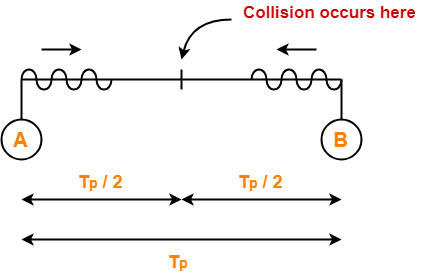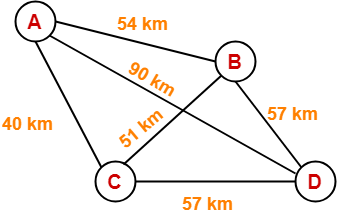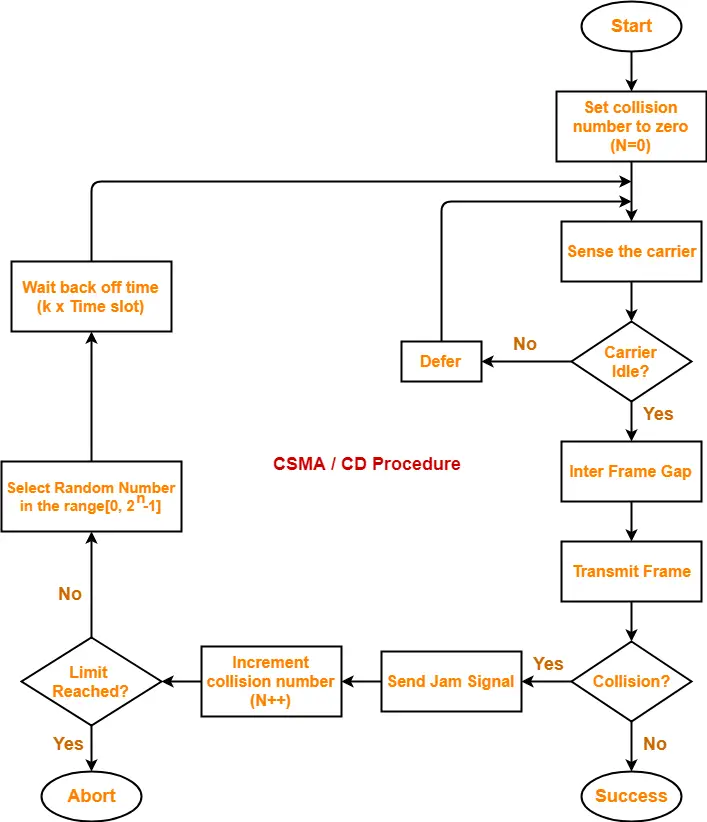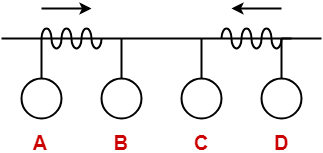## CSMA / CD Protocol-

Before you go through this article, make sure that you have gone through the previous article on CSMA / CD Protocol.

We have discussed-

• CSMA / CD allows a station to transmit data if it senses the carrier free.
• After undergoing collision, station waits for random back off time before transmitting again.
• Back Off Algorithm is used to calculate back off time.

In this article, we will discuss practice problems based on CSMA / CD and Back Off Algorithm.

## Problem-01:

After the kth consecutive collision, each colliding station waits for a random time chosen from the interval-

1. (0 to 2k) x RTT
2. (0 to 2k-1) x RTT
3. (0 to 2k-1) x Maximum Propagation delay
4. (0 to 2k-1) x Maximum Propagation delay

## Solution-

Clearly, Option (B) is correct.

## Problem-02:

In a CSMA / CD network running at 1 Gbps over 1 km cable with no repeaters, the signal speed in the cable is 200000 km/sec. What is minimum frame size?

## Solution-

Given-

• Bandwidth = 1 Gbps
• Distance = 1 km
• Speed = 200000 km/sec

### Calculating Propagation Delay-

Propagation delay (Tp)

= Distance / Propagation speed

= 1 km / (200000 km/sec)

= 0.5 x 10-5 sec

= 5 x 10-6 sec

### Calculating Minimum Frame Size-

Minimum frame size

= 2 x Propagation delay x Bandwidth

= 2 x 5 x 10-6 sec x 109 bits per sec

= 10000 bits

## Problem-03:

A 2 km long broadcast LAN has 107 bps bandwidth and uses CSMA / CD. The signal travels along the wire at 2 x 108 m/sec. What is the minimum packet size that can be used on this network?

1. 50 B
2. 100 B
3. 200 B
4. None of the above

## Solution-

Given-

• Distance = 2 km
• Bandwidth = 107 bps
• Speed = 2 x 108 m/sec

### Calculating Propagation Delay-

Propagation delay (Tp)

= Distance / Propagation speed

= 2 km / (2 x 108 m/sec)

= 2 x 103 m / (2 x 108 m/sec)

= 10-5 sec

### Calculating Minimum Frame Size-

Minimum frame size

= 2 x Propagation delay x Bandwidth

= 2 x 10-5 sec x 107 bits per sec

= 200 bits or 25 bytes

Thus, Option (D) is correct.

## Problem-04:

A and B are the only two stations on Ethernet. Each has a steady queue of frames to send. Both A and B attempts to transmit a frame, collide and A wins first back off race. At the end of this successful transmission by A, both A and B attempt to transmit and collide. The probability that A wins the second back off race is ___ .

1. 0.5
2. 0.625
3. 0.75
4. 1.0

## Solution-

According to question, we have-

### 1st Transmission Attempt-

• Both the stations A and B attempts to transmit a frame.
• A collision occurs.
• Back Off Algorithm runs.
• Station A wins and successfully transmits its 1st data packet.

### 2nd Transmission Attempt-

• Station A attempts to transmit its 2nd data packet.
• Station B attempts to retransmit its 1st data packet.
• A collision occurs.

Now,

• We have been asked the probability of station A to transmit its 2nd data packet successfully after 2nd collision.
• After the 2nd collision occurs, we have-

### At Station A-

• 2nd data packet of station A undergoes collision for the 1st time.
• So, collision number for the 2nd data packet of station A = 1.
• Now, station A randomly chooses a number from the range [0,21-1] = [0,1].
• Then, station A waits for back off time and then attempts to retransmit its data packet.

### At Station B-

• 1st data packet of station B undergoes collision for the 2nd time.
• So, collision number for the 1st data packet of station B = 2.
• Now, station B randomly chooses a number from the range [0,22-1] = [0,3].
• Then, station B waits for back off time and then attempts to retransmit its data packet.

Following 8 cases are possible-

 Station A Station B Remark 0 0 Collision 0 1 A wins 0 2 A wins 0 3 A wins 1 0 B wins 1 1 Collision 1 2 A wins 1 3 A wins

From here,

• Probability of A winning the 2nd back off race = 5 / 8 = 0.625.
• Thus, Option (B) is correct.

## Problem-05:

Suppose nodes A and B are on same 10 Mbps Ethernet segment and the propagation delay between two nodes is 225 bit times. Suppose A and B send frames at t=0, the frames collide then at what time, they finish transmitting a jam signal. Assume a 48 bit jam signal.

## Solution-

Propagation delay (Tp)

= 225 bit times

= 225 bit / 10 Mbps

= 22.5 x 10-6 sec

= 22.5 μsec

### At t = 0,

• Nodes A and B start transmitting their frame.
• Since both the stations start simultaneously, so collision occurs at the mid way.
• Time after which collision occurs = Half of propagation delay.
• So, time after which collision occurs = 22.5 μsec / 2 = 11.25 μsec.### At t = 11.25 μsec,

• After collision occurs at t = 11.25 μsec, collided signals start travelling back.
• Collided signals reach the respective nodes after time = Half of propagation delay
• Collided signals reach the respective nodes after time = 22.5 μsec / 2 = 11.25 μsec.
• Thus, at t = 22.5 μsec, collided signals reach the respective nodes.

### At t = 22.5 μsec,

• As soon as nodes discover the collision, they immediately release the jam signal.
• Time taken to finish transmitting the jam signal = 48 bit time = 48 bits/ 10 Mbps = 4.8 μsec.

Thus,

Time at which the jam signal is completely transmitted

= 22.5 μsec + 4.8 μsec

= 27.3 μsec or 273 bit times

## Problem-06:

Suppose nodes A and B are attached to opposite ends of the cable with propagation delay of 12.5 ms. Both nodes attempt to transmit at t=0. Frames collide and after first collision, A draws k=0 and B draws k=1 in the exponential back off protocol. Ignore the jam signal. At what time (in seconds), is A’s packet completely delivered at B if bandwidth of the link is 10 Mbps and packet size is 1000 bits.

## Solution-

Given-

• Propagation delay = 12.5 ms
• Bandwidth = 10 Mbps
• Packet size = 1000 bits

### Time At Which Collision Occurs-

Collision occurs at the mid way after time

= Half of Propagation delay

= 12.5 ms / 2

= 6.25 ms

Thus, collision occurs at time t = 6.25 ms.

### Time At Which Collision is Discovered-

Collision is discovered in the time it takes the collided signals to reach the nodes

= Half of Propagation delay

= 12.5 ms / 2

= 6.25 ms

Thus, collision is discovered at time t = 6.25 ms + 6.25 ms = 12.5 ms.

### Scene After Collision-

After the collision is discovered,

• Both the nodes wait for some random back off time.
• A chooses k=0 and then waits for back off time = 0 x 25 ms = 0 ms.
• B chooses k=1 and then waits for back off time = 1 x 25 ms = 25 ms.
• From here, A begins retransmission immediately while B waits for 25 ms.

### Waiting Time For A-

• After winning the back off race, node A gets the authority to retransmit immediately.
• But node A does not retransmit immediately.
• It waits for the channel to clear from the last bit aborted by it on discovering the collision.
• Time taken by the last bit to get off the channel = Propagation delay = 12.5 ms.
• So, node A waits for time = 12.5 ms and then starts the retransmission.
• Thus, node A starts the retransmission at time t = 12.5 ms + 12.5 ms = 25 ms.

### Time Taken in Delivering Packet To Node B-

Time taken to deliver the packet to node B

= Transmission delay + Propagation delay

= (1000 bits / 10 Mbps) + 12.5 ms

= 100 μs + 12.5 ms

= 0.1 ms + 12.5 ms

= 12.6 ms

Thus, At time t = 25 ms + 12.6 ms = 37.6 ms, the packet is delivered to node B.

## Problem-07:

The network consists of 4 hosts distributed as shown below-Assume this network uses CSMA / CD and signal travels with a speed of 3 x 105 km/sec. If sender sends at 1 Mbps, what could be the minimum size of the packet?

1. 600 bits
2. 400 bits
3. 6000 bits
4. 1500 bits

## Solution-

• CSMA / CD is a Access Control Method.
• In the given network, all the links are point to point.
• So, there is actually no need of implementing CSMA / CD.
• Stations can transmit whenever they want to transmit.

 In CSMA / CD,The condition to detect collision is-Packet size >= 2 x (distance / speed) x Bandwidth

To solve the question,

• We assume that a packet of same length has to be used in the entire network.
• To get the minimum length of the packet, what distance we should choose?
• To get the minimum length of the packet, we should choose the minimum distance.
• But, then collision would be detected only in the links having distance less than or equal to that minimum distance.
• For the links, having distance greater than the minimum distance, collision would not be detected.
• So, we choose the maximum distance so that collision can be detected in all the links of the network.

So, we use the values-

• Distance = 90 km
• Speed = 3 x 105 km/sec
• Bandwidth = 1 Mbps

Substituting these values, we get-

Minimum size of data packet

= 2 x (90 km / 3 x 105 km per sec) x 1 Mbps

= 2 x 30 x 10-5 sec x 106 bits per sec

= 600 bits

Thus, Option (A) is correct.

Next Article- Token Passing | Access Control Method

Get more notes and other study material of Computer Networks.

Watch video lectures by visiting our YouTube channel LearnVidFun.

## CSMA / CD Protocol-

Before you go through this article, make sure that you have gone through the previous article on CSMA / CD Protocol.

We have discussed-

• CSMA / CD stands for Carrier Sense Multiple Access / Collision Detection.
• It allows the stations to sense the carrier and transmit data if the carrier is free.

The following CSMA / CD flowchart shows the CSMA / CD procedure-## Back Off Time-

In CSMA / CD protocol,

• After the occurrence of collision, station waits for some random back off time and then retransmits.
• This waiting time for which the station waits before retransmitting the data is called as back off time.
• Back Off Algorithm is used for calculating the back off time.

## Back Off Algorithm-

After undergoing the collision,

• Transmitting station chooses a random number in the range [0, 2n-1] if the packet is undergoing collision for the nth time.
• If station chooses a number k, then-

 Back off time = k x Time slot

where value of one time slot = 1 RTT

## Example-

Consider the following scenario where stations A and D start transmitting their data simultaneously-For simplicity,

• We consider the value of time slot = 1 unit.
• Thus, back off time = K units.

## Scene-01: For 1st Data Packet Of Both Stations-

• Both the stations start transmitting their 1st data packet simultaneously.
• This leads to a collision.
• Clearly, the collision on both the packets is occurring for the 1st time.
• So, collision number for the 1st data packet of both the stations = 1.

## At Station A-

After detecting the collision,

• Station A randomly chooses a number in the range [0, 21-1] = [0,1].
• If station A chooses the number KA, then back off time = KA units.

## At Station D-

After detecting the collision,

• Station D randomly chooses a number in the range [0, 21-1] = [0,1].
• If station D chooses the number KD, then back off time = KD units.

Following 4 cases are possible-

 KA KD Remarks 0 0 In this case, both the stations start retransmitting their data immediately.This case leads to a collision again. 0 1 In this case, station A starts retransmitting its data immediately while station D waits for 1 unit of time.This case leads to A successfully retransmitting its data after the 1st collision. 1 0 In this case, station A waits for 1 unit of time while station D starts retransmitting its data immediately.This case leads to D successfully retransmitting its data after the 1st collision. 1 1 In this case, both the stations wait for 1 unit of time and then starts retransmitting their data simultaneously.This case leads to a collision again.

From here,

• Probability of station A to successfully retransmit its data after the 1st collision = 1 / 4
• Probability of station D to successfully retransmit its data after the 1st collision = 1 / 4
• Probability of occurrence of collision again after the 1st collision = 2 / 4 = 1 / 2

Now,

• Consider case-02 occurs.
• This causes station A to successfully retransmit its 1st packet after the 1st collision.

## Scene-02: For 2nd Data Packet Of Station A And 1st Data Packet Of Station D-

Consider after some time,

• Station A starts transmitting its 2nd data packet and station D starts retransmitting its 1st data packet simultaneously.
• This leads to a collision.

## At Station A-

• The 2nd data packet of station A undergoes collision for the 1st time.
• So, collision number for the 2nd data packet of station A = 1.
• Now, station A randomly chooses a number in the range [0, 21-1] = [0,1].
• If station A chooses the number KA, then back off time = KA units.

## At Station D-

• The 1st data packet of station D undergoes collision for the 2nd time.
• So, collision number for the 1st data packet of station D = 2.
• Now, station D randomly chooses a number in the range [0, 22-1] = [0,3].
• If station D chooses the number KD, then back off time = KD units.

Following 8 cases are possible-

 KA KD Remarks 0 0 In this case, both the stations start retransmitting their data immediately.This case leads to a collision again. 0 1 In this case, station A starts retransmitting its data immediately while station D waits for 1 unit of time.This case leads to A successfully retransmitting its data after the 2nd collision. 0 2 In this case, station A starts retransmitting its data immediately while station D waits for 2 unit of time.This case leads to A successfully retransmitting its data after the 2nd collision. 0 3 In this case, station A starts retransmitting its data immediately while station D waits for 3 unit of time.This case leads to A successfully retransmitting its data after the 2nd collision. 1 0 In this case, station A waits for 1 unit of time while station D starts retransmitting its data immediately.This case leads to D successfully retransmitting its data after the 2nd collision. 1 1 In this case, both the stations wait for 1 unit of time and then starts retransmitting their data simultaneously.This case leads to a collision again. 1 2 In this case, station A waits for 1 unit of time while station D waits for 2 unit of time.This case leads to A successfully retransmitting its data after the 2nd collision. 1 3 In this case, station A waits for 1 unit of time while station D waits for 3 unit of time.This case leads to A successfully retransmitting its data after the 2nd collision.

From here,

• Probability of station A to successfully retransmit its data after the 2nd collision = 5 / 8
• Probability of station D to successfully retransmit its data after the 2nd collision = 1 / 8
• Probability of occurrence of collision again after the 2nd collision = 2 / 8 = 1 / 4

Now,

• Consider case-03 occurs.
• This causes station A to successfully retransmit its 2nd packet after the 2nd collision.

## Scene-03: For 3rd Data Packet Of Station A And 1st Data Packet Of Station D-

Consider after some time,

• Station A starts transmitting its 3rd data packet and station D starts retransmitting its 1st data packet simultaneously.
• This leads to a collision.

## At Station A-

• The 3rd data packet of station A undergoes collision for the 1st time.
• So, collision number for the 3rd data packet of station A = 1.
• Now, station A randomly chooses a number in the range [0, 21-1] = [0,1].
• If station A chooses the number KA, then back off time = KA unit.

## At Station D-

• The 1st data packet of station D undergoes collision for the 3rd time.
• So, collision number for the 1st data packet of station D = 3.
• Now, station D randomly chooses a number in the range [0, 23-1] = [0,7].
• If station D chooses the number KD, then back off time = KD unit.

Following 16 cases are possible-

From here,

• Probability of station A to successfully retransmit its data after the 3rd collision = 13 / 16
• Probability of station D to successfully retransmit its data after the 3rd collision = 1 / 16
• Probability of occurrence of collision again after the 3rd collision = 1 / 16

In the similar manner, the procedure continues.

## Note-01:

With each successive collision-

• Back off time increases exponentially.
• Collision probability decreases exponentially.

## Note-02:

Back Off Algorithm is also known as Binary Exponential Back Off Algorithm because-

• It works for only two stations.
• The back off time increases exponentially.
• Collision probability decreases exponentially.

## Note-03:

• One disadvantage of Back Off Algorithm is that it shows capture effect.
• It means if a particular station wins the collision one time, then its probability of winning the successive collisions increases exponentially.

To gain better understanding about Back Off Algorithm,

Watch this Video Lecture

Next Article- Practice Problems On CSMA / CD & Back Off Algorithm

Get more notes and other study material of Computer Networks.

Watch video lectures by visiting our YouTube channel LearnVidFun.